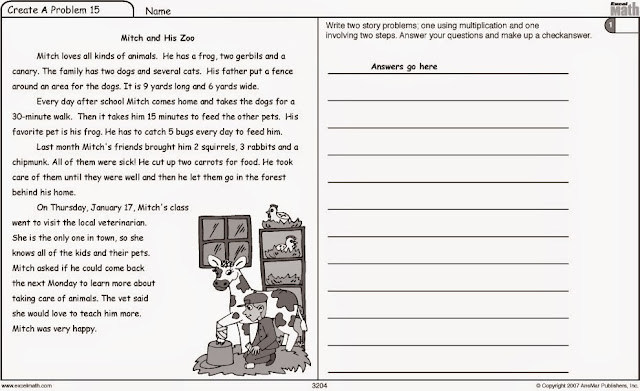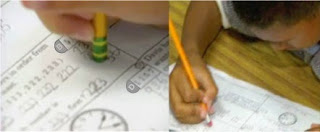## Monday, December 4, 2017

### Giving Students Feedback in Your Math ClassNew math standards are asking teachers to facilitate discussions and encourage collaborative problem solving among students in the math classroom.

Giving students effective feedback and helping them to "explain their thinking" will let you determine their depth of understanding. It will also give you a chance to clear up any misconceptions before moving on to more difficult concepts.

Excel Math lessons have discussion questions and teaching tips built into the Teacher Edition for Kindergarten through Grade Six.

Create A Problem Exercises begin with Grade 2 and provide a template for students to write and solve their own word problems, merging math with literacy. In Excel Math we suggest the teacher help students verbalize the problem-solving strategies they use as they solve problems.Create A Problem from Excel Math lesson sheets for Grade 3

Describing the process can help their classmates understand that at times there can be several correct ways to solve one problem. Remind your students to show their work as they solve the problem so if they have errors in the solution, they can find and fix them more easily.Explain that making mistakes and correcting them is the way to improve and learn. Excel Math includes a unique CheckAnswer system beginning in Grade 2 that lets students check their own work. Most of the time, students can find and correct their mistakes on their own.

When they need more help, a parent or teacher can help them work through the problem to find the correct answer.

Here are three steps to giving students effective feedback:
1. Ask if they understand the question or problem:
a. What information are they trying to obtain? Have them circle the question.

b. What information have they been given? Have them draw a line under the information that will be needed and cross out the information that will not be needed.

c. Do they have the information that they need to get the answer? Find out if the information they have is enough to answer the question.
2. If there is not enough information, ask the student to tell you what information they would need to answer the question or solve the problem.

3. If there is enough information, ask the student to tell you if they underlined the correct information that will be needed. Did they underline too much or little information to solve the problem? If so, have them fix it.After following these three steps with your students, try using questions and statements rather giving answers when someone is having trouble remembering what to do to solve a math problem. You could ask:
What do you think you need to do first to find the answer?
Do you understand what the question is asking? Tell me in your own words. (This is a good place to make sure any vocabulary words used in the problem are clearly defined and understood.)

What steps should you take to find the solution?

Show me how you figured that out.

Tell me about the problem-solving strategy you used.

What other ways could you have solved this problem?Take a look at the strategies you use to give your students feedback.

Do any of them need to be modified? Could you use the buddy system to have students help each other find the solutions? Share your suggestions in the Comments box below.

New to Excel Math? Preview elementary math lessons that really work for Kindergarten through Sixth Grade on our website: www.excelmath.com

Also find math resources for teachers, parents and students and download a sample packet at excelmath.com.